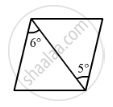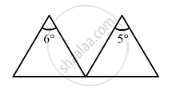Department of Pre-University Education, KarnatakaPUC Karnataka Science Class 12
Advertisement Remove all ads

# A Thin Prism of Angle 6.0°, ω = 0.07 and μY = 1.50 is Combined with Another Thin Prism Having ω = 0.08 and μY = 1.60. the Combination Produces No Deviation in the Mean Ray. - Physics

Sum

A thin prism of angle 6.0°, ω = 0.07 and μy = 1.50 is combined with another thin prism having ω = 0.08 and μy = 1.60. The combination produces no deviation in the mean ray. (a) Find the angle of the second prism. (b) Find the net angular dispersion produced by the combination when a beam of white light passes through it. (c) If the prisms are similarly directed, what will be the deviation in the mean ray? (d) Find the angular dispersion in the situation described in (c).

Advertisement Remove all ads

#### Solution

Given:-

For the first prism,

Angle of prism, A' = 6°

Angle of deviation, ω' = 0.07

Refractive index for yellow colour, μ'y = 1.50

For the second prism,

Angle of deviation, ω = 0.08

Refractive index for yellow colour, μy= 1.60

Let the angle of prism for the second prism be A.

The prism must be oppositely directed, as the combination produces no deviation in the mean ray.

(a) The deviation of the mean ray is zero.

Thus, we have:-

δy = (μy – 1)A – (μ'y – 1)A' = 0

∴ (1.60 – 1)A = (1.50 – 1)A'

rArrA=(0.50xx6^o)/0.60=5^o(b) Net angular dispersion on passing a beam of white light:-

y – 1)ωA – (μy – 1)ω'A'

⇒ (1.60 – 1)(0.08)(5°) – (1.50 – 1)(0.07)(6°)

⇒ 0.24° – 0.21° = 0.03°(c) For the prisms directed similarly, the net deviation in the mean ray is given by

δy = (μy – 1)A + (μy – 1)A'
= (1.60 – 1)5° + (1.50 – 1)6°
= 3° + 3° = 6°

(d) For the prisms directed similarly, angular dispersion is given by

δv – δr = (μy – 1)ωA – (μy – 1)ω'A'
= 0.24° + 0.21°
= 0.45°

Is there an error in this question or solution?
Advertisement Remove all ads

#### APPEARS IN

HC Verma Class 11, 12 Concepts of Physics 1
Chapter 20 Dispersion and Spectra
Q 10 | Page 443
Advertisement Remove all ads

#### Video TutorialsVIEW ALL 

Advertisement Remove all ads
Share
Notifications

View all notifications

Forgot password?